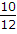# Electronics and Communication Engineering - Digital Electronics

36.

Which display device resembles vacuum tube?

 A. LED B. LCD C. VF D. None of these

Explanation:

It is similar to triode.

37.

The number of inputs and outputs of a full adder are

 A. 3 and 2 respectively B. 2 and 3 respectively C. 4 and 2 respectively D. 2 and 4 respectively

Explanation:

Inputs are carry from lower bits and two other bits. Outputs are SUM and CARRY.

38.

A VF display operates on the principle of a vacuum diode.

 A. True B. False

Explanation:

It operates on the principle of vacuum triode.

39.

In a 3 input NAND gate, the number of states in which output is 0 equals

 A. 8 B. 1 C. 6 D. 5

Explanation:

Only one input, i.e., A = 1, B = 1 and C = 1 gives low output.

40.

In a mod-12 counter the input clock frequency is 10 kHz. The output frequency is

 A. 0.833 kHz B. 1.0 kHz C. 0.91 kHz D. 0.77 kHz

Mod-12 counter is divide by 12 counter. Output frequency == 0.833 kHz.